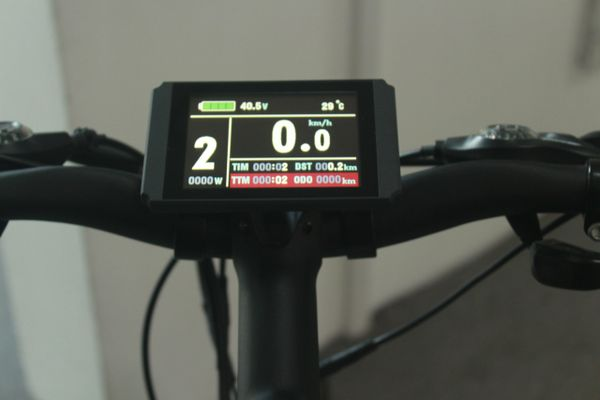# Color KT-LCD8H 60v

\$99.99

Color KT-LCD8H 60v is for a 60v battery.

This unit is compatible with our 35a 60v KT controller.

Package will include the LCD, long cable with a green HIGO connector and mounting bracket with hardware.

P1 = 100

P2 = 5

P3 = 1

P4 = 0

P5 = 0

C1 = 3

C2 = 0

C3 = 1

C4 = 0

C5 = 2

C6 = 3

C7 = 1

C8 = 0

C9 = 0

C10 = n

C11 = 0

C12 = 4

C13 = 0

C14 = 1

L1=0

L2=0

L3=1

L4=5